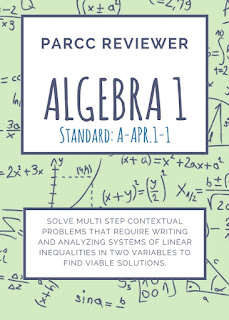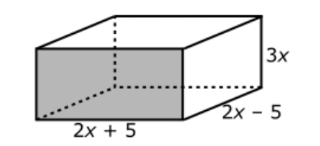## Labels

### Algebra 1 PARCC Reviewer 1 (A-APR.1-1)

Sub-claim: A
The students solve problems involving the Major Content with connections to the Standards for Mathematical Practice
Type of items: Type 1
Color Code: Peach
Evidence Statement Key: A-APR.1-1
Calculator: Z
Y = Yes; Assessed on Calculator Section
X = Calculator is Specific to Item
N = No; Assessed on Non-Calculator Sections
Z = Calculator Neutral (Could be Calculator or Non-Calculator Sections)
Point: 1 each (1:26)
Evidence Statement Text:
Clarifications, limits, emphases, and other information intended to ensure appropriate variety in tasks:
i) The "understand" part of the standard is not assessed here; it is assessed under Sub-claim C.
Sample Questions (taken from PARCC’s Practice Tests and Released Items):
EOY Released Test Item 10
PBA Released Test Item 5
Practice Test, Unit 1, Item 5
Practice Test, Unit 1, Item 4
2016 #4
2015 Fall Block #21.EOY Released Test Item #10

The diagram represents a right rectangular prism with dimensions labeled as algebraic expressions. Which of these expressions represents the volume of the prism? Select all that apply.a. 7x

b. 4x2 - 25
c. 12x- 75x
d. 3x (4x- 25)
e. (2x + 5) (6x- 15x)
f. 12x+ 60x3 + 75x
g. 12x- 30x3 + 10x - 25

2. PBA Released Test Item #5
Multiply the polynomials ( x + 3 ) ( 2x - 4 ). What is the product in the form
a = _____
b = _____
c = _____

3. Practice Test, Unit 1, Item 5
Which points are on the graph of the equation -3x + 6y + 5 = -7?
Select all that apply.
a. ( -3 , 6 )
b. ( -2 , 0 )
c. ( 0 , -2 )
d. ( 6 , -3 )
e. ( 8 , 2 )

4. Practice Test, Unit 1, Item 4
Which expression is equivalent to (3x5 + 8x3) - (7x2 - 6x3)?
a. -4x3 + 14
b. -4x5 + 14x3
c. 3x5 + 14x3 - 7x2
d. 3x5 + 2x3 - 7x2

5. 2016 #4

The polynomial (2x - 1) (x2 - 2) - x(x2 - x - 2) can be written in the form
ax3 + bx2 + cx + d, where a, b, c, and d are constants. What are the values of a, b, c, and d? Select from the choices listed below to correctly complete each value.
-2
-1
0
1
2

a = _______
b = _______
c = _______
d = _______

6. 2015 Fall Block #2

Consider the polynomial expression: x4 - 8x2 + 16.
Select all expressions that are equivalent to the given expression.
a. (x2 - 8) (x2 - 2)
b. (x2 - 4)2
c. 8 - 3x4 + x2 + 4x4 + 8 - 9x2
d. - (-16 - 8x2 - x4 )
e. (x3+ x) - (6x + 2x) + 16